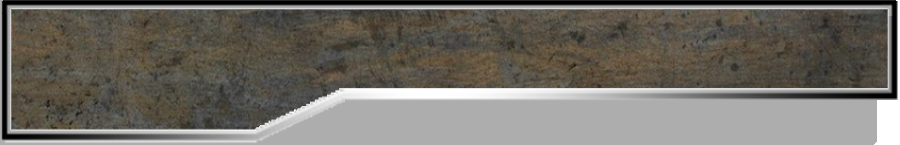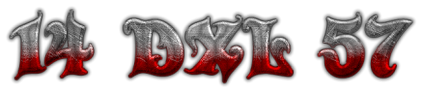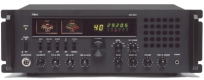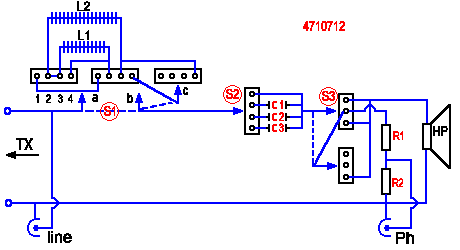Schematic diagram :List of components :
S1 = Rotary switch 4 positions 3 circuits ( connect point 2 and 3 )
S2 = Rotary switch 4 positions 1 circuits
S3 = Rotary switch 3 positions 3 circuits ( HP alone, Phone alone or both )
C1 = 10 microFarad - C2 = 4.7 microFarad - C3 = 2.2 microFarad
L1 = 0.5 mH - L2 = 1.2 mH ( Self-air filter type for loudspeaker )
R1 = 68 Homs 5 Watt, - R2 = 100 Homs 5 Watt
HP = Speaker 8 Homs